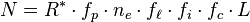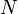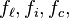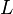# Drake equation facts for kids

Kids Encyclopedia Facts

In 1961, Frank Drake wrote down an equation for the chance of a contactable alien civilization from another planet in the Milky Way Galaxy. This is known as the Drake Equation (sometimes called the Green Bank equation or the Greeen Bank Formula). Carl Sagan mentioned the Drake equation often so it that has been mistaken for the 'Sagan equation'.

## The equation

The Drake equation states that:$N = R^{\ast} \cdot f_p \cdot n_e \cdot f_{\ell} \cdot f_i \cdot f_c \cdot L \!$

where:

N = the number of civilizations in our galaxy with which communication might be possible;

and

R* = the average rate of star formation per year in our galaxy
fp = the fraction of those stars that have planets
net= the average number of planets that can potentially support life per star that has planets
f = the fraction of the above that actually go on to develop life at some point
fi = the fraction of the above that actually go on to develop intelligent life
fc = the fraction of civilizations that develop a technology that releases detectable signs of their existence into space
L = the length of time for which such civilizations release detectable signals into space.

## Solving it

We don't know the solution to the equation.

Although written as an equation, Drake's formulation is not particularly useful for getting a value of$N$. The last four parameters,$f_{\ell}, f_i, f_c,$ and$L$ are not known. They are very hard to guess, with values ranging over many orders of magnitude. Therefore, the SETI League says the importance of the Drake equation is not in solving it, but in thinking about it. It may be more useful to think of it as a series of questions framed as a numbers game.

## Images for kidsDrake equation Facts for Kids. Kiddle Encyclopedia.